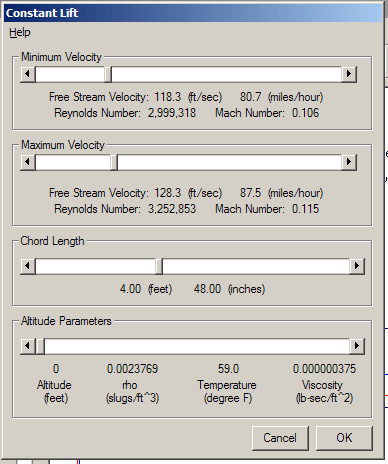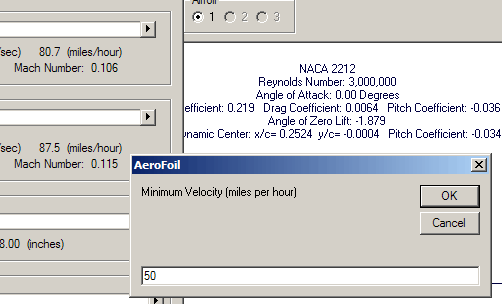## Constant LiftFor an airplane in stable flight, angle of attack determines the airspeed. Boundary layer development and maximum lift coefficient is highly dependent upon the Reynolds number, which is a function of the airspeed, airfoil length, air density, and air viscosity.

The Constant Lift option assumes the value given by 0.5 * ρ * V2 * Lift Coefficient is constant as a function of the angle of attack.

The program user must specify a minimum and maximum velocity range over which the calculation will be performed, the airfoil chord, and the air parameters.

AeroFoil will calculate the angle of attack at the specified minimum velocity through iteration. The lowest angle of attack is then calculated that will give the same section lift at the maximum velocity. The range of angle of attack is sub-divided into equal segments. At each new angle of attack, the process is repeated such that the section lift remains constant.Any of the free stream velocities, either chord length, and the altitude values may be entered by left clicking over the numeric value and typing the desired value in the text box.